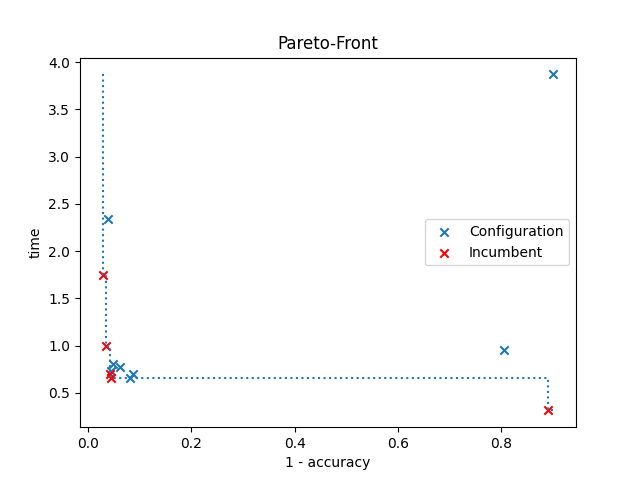# ParEGO¶

An example of how to use multi-objective optimization with ParEGO. Both accuracy and run-time are going to be optimized on the digits dataset using an MLP, and the configurations are shown in a plot, highlighting the best ones in a Pareto front. The red cross indicates the best configuration selected by SMAC.

In the optimization, SMAC evaluates the configurations on two different seeds. Therefore, the plot shows the mean accuracy and run-time of each configuration.```[WARNING][target_function_runner.py:72] The argument budget is not set by SMAC: Consider removing it from the target function.
[INFO][abstract_initial_design.py:147] Using 5 initial design configurations and 0 additional configurations.
[INFO][abstract_intensifier.py:515] Added config 3623cc as new incumbent because there are no incumbents yet.
[INFO][abstract_intensifier.py:598] Config 540db2 is a new incumbent. Total number of incumbents: 2.
[INFO][abstract_intensifier.py:598] Config 3b5efd is a new incumbent. Total number of incumbents: 3.
[INFO][abstract_intensifier.py:598] Config a464c2 is a new incumbent. Total number of incumbents: 4.
[INFO][abstract_intensifier.py:598] Config fa4572 is a new incumbent. Total number of incumbents: 5.
[INFO][abstract_intensifier.py:598] Config 63b370 is a new incumbent. Total number of incumbents: 4.
[INFO][abstract_intensifier.py:598] Config f262a9 is a new incumbent. Total number of incumbents: 5.
[INFO][smbo.py:327] Configuration budget is exhausted:
[INFO][smbo.py:328] --- Remaining wallclock time: -0.5777037143707275
[INFO][smbo.py:329] --- Remaining cpu time: inf
[INFO][smbo.py:330] --- Remaining trials: 175
Validated costs from default config:
--- [0.60155834 0.24215281]

Validated costs from the Pareto front (incumbents):
--- [0.88924791 0.29182327]
--- [0.03561281 1.11070251]
--- [0.04396781 0.72936821]
--- [0.04229805 0.75202131]
--- [0.02838672 1.71442103]
```

```from __future__ import annotations

import time
import warnings

import matplotlib.pyplot as plt
import numpy as np
from ConfigSpace import (
Categorical,
Configuration,
ConfigurationSpace,
EqualsCondition,
Float,
InCondition,
Integer,
)
from sklearn.model_selection import StratifiedKFold, cross_val_score
from sklearn.neural_network import MLPClassifier

from smac import Scenario
from smac.multi_objective.parego import ParEGO

class MLP:
@property
def configspace(self) -> ConfigurationSpace:
cs = ConfigurationSpace()

n_layer = Integer("n_layer", (1, 5), default=1)
n_neurons = Integer("n_neurons", (8, 256), log=True, default=10)
activation = Categorical("activation", ["logistic", "tanh", "relu"], default="tanh")
batch_size = Integer("batch_size", (30, 300), default=200)
learning_rate = Categorical("learning_rate", ["constant", "invscaling", "adaptive"], default="constant")
learning_rate_init = Float("learning_rate_init", (0.0001, 1.0), default=0.001, log=True)

cs.add_hyperparameters([n_layer, n_neurons, activation, solver, batch_size, learning_rate, learning_rate_init])

use_lr = EqualsCondition(child=learning_rate, parent=solver, value="sgd")
use_lr_init = InCondition(child=learning_rate_init, parent=solver, values=["sgd", "adam"])
use_batch_size = InCondition(child=batch_size, parent=solver, values=["sgd", "adam"])

# We can also add multiple conditions on hyperparameters at once:

return cs

def train(self, config: Configuration, seed: int = 0, budget: int = 10) -> dict[str, float]:
lr = config.get("learning_rate", "constant")
lr_init = config.get("learning_rate_init", 0.001)
batch_size = config.get("batch_size", 200)

start_time = time.time()

with warnings.catch_warnings():
warnings.filterwarnings("ignore")

classifier = MLPClassifier(
hidden_layer_sizes=[config["n_neurons"]] * config["n_layer"],
solver=config["solver"],
batch_size=batch_size,
activation=config["activation"],
learning_rate=lr,
learning_rate_init=lr_init,
max_iter=int(np.ceil(budget)),
random_state=seed,
)

# Returns the 5-fold cross validation accuracy
cv = StratifiedKFold(n_splits=5, random_state=seed, shuffle=True)  # to make CV splits consistent
score = cross_val_score(classifier, digits.data, digits.target, cv=cv, error_score="raise")

return {
"1 - accuracy": 1 - np.mean(score),
"time": time.time() - start_time,
}

def plot_pareto(smac: AbstractFacade, incumbents: list[Configuration]) -> None:
"""Plots configurations from SMAC and highlights the best configurations in a Pareto front."""
average_costs = []
average_pareto_costs = []
for config in smac.runhistory.get_configs():
# Since we use multiple seeds, we have to average them to get only one cost value pair for each configuration
average_cost = smac.runhistory.average_cost(config)

if config in incumbents:
average_pareto_costs += [average_cost]
else:
average_costs += [average_cost]

# Let's work with a numpy array
costs = np.vstack(average_costs)
pareto_costs = np.vstack(average_pareto_costs)
pareto_costs = pareto_costs[pareto_costs[:, 0].argsort()]  # Sort them

costs_x, costs_y = costs[:, 0], costs[:, 1]
pareto_costs_x, pareto_costs_y = pareto_costs[:, 0], pareto_costs[:, 1]

plt.scatter(costs_x, costs_y, marker="x", label="Configuration")
plt.scatter(pareto_costs_x, pareto_costs_y, marker="x", c="r", label="Incumbent")
plt.step(
[pareto_costs_x] + pareto_costs_x.tolist() + [np.max(costs_x)],  # We add bounds
[np.max(costs_y)] + pareto_costs_y.tolist() + [np.min(pareto_costs_y)],  # We add bounds
where="post",
linestyle=":",
)

plt.title("Pareto-Front")
plt.xlabel(smac.scenario.objectives)
plt.ylabel(smac.scenario.objectives)
plt.legend()
plt.show()

if __name__ == "__main__":
mlp = MLP()
objectives = ["1 - accuracy", "time"]

# Define our environment variables
scenario = Scenario(
mlp.configspace,
objectives=objectives,
walltime_limit=30,  # After 30 seconds, we stop the hyperparameter optimization
n_trials=200,  # Evaluate max 200 different trials
n_workers=1,
)

# We want to run five random configurations before starting the optimization.
multi_objective_algorithm = ParEGO(scenario)

# Create our SMAC object and pass the scenario and the train method
scenario,
mlp.train,
initial_design=initial_design,
multi_objective_algorithm=multi_objective_algorithm,
intensifier=intensifier,
overwrite=True,
)

# Let's optimize
incumbents = smac.optimize()

# Get cost of default configuration
default_cost = smac.validate(mlp.configspace.get_default_configuration())
print(f"Validated costs from default config: \n--- {default_cost}\n")

print("Validated costs from the Pareto front (incumbents):")
for incumbent in incumbents:
cost = smac.validate(incumbent)
print("---", cost)

# Let's plot a pareto front
plot_pareto(smac, incumbents)
```

Total running time of the script: ( 0 minutes 40.514 seconds)